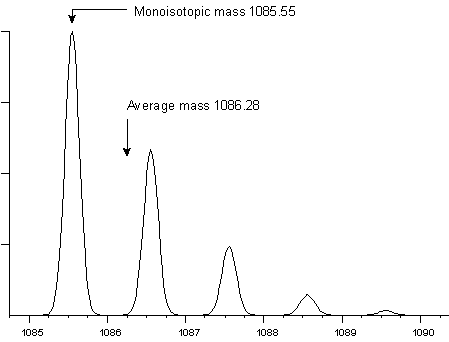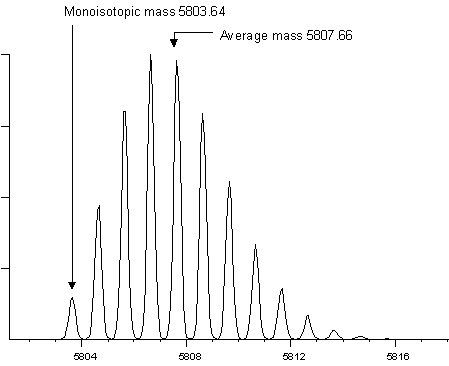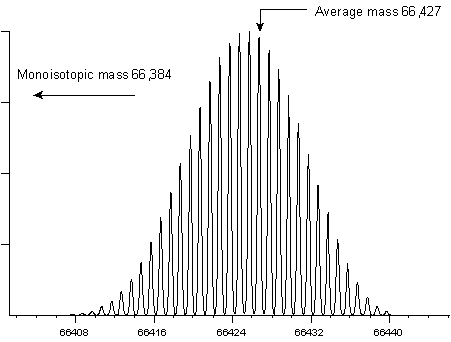# Accuracy & resolution

### Types of Mass Measurement

When low molecular weight samples are being analysed using relatively low resolution mass spectrometers, it is common to work with "nominal" mass values, calculated from integer atomic weights. That is, H=1, C=12, N=14, O=16, etc. Nominal mass is rarely used in peptide and protein work because the cumulative error of approximating atomic weights with integers becomes unacceptable.

The presence of isotopes at their natural abundances makes it essential to define whether an experimental mass value is an "average" value, equivalent to taking the centroid of the complete isotopic envelope, or a "monoisotopic" value, the mass of the first peak of the isotope distribution.

For peptides and proteins, the difference between an average and a monoisotopic weight is approximately 0.06%. This is a significant difference when even the most modest instruments are capable of measuring the mass of a small peptide with an accuracy of a fraction of a Dalton. For example, peptide HLKTEAEMK has an average molecular weight of 1086.28 and a monoisotopic weight of 1085.55. At a mass resolution of 5000, the isotopic envelope has this appearance:Mass resolution is the dimensionless ratio of the mass of the peak divided by its width. Usually, the peak width is taken as the full width at half maximum intensity, (fwhm). However, this definition of peak width is only a convention, and you may also encounter data acquired on magnetic sector instruments where the resolution has been calculated using the peak width at 5% maximum intensity.

To measure a monoisotopic molecular weight requires (i) sufficient mass resolution to resolve the isotopic distribution (ii) sufficient signal to noise to be able to identify the first peak of the envelope with confidence. For a small peptide, the first peak (often referred to as the 12C peak) is also the most intense peak. This is not the case for larger molecules. The following two examples show the isotopic envelopes for a small protein (insulin) and a larger protein (BSA):It would be extremely difficult to measure a monoisotopic mass for BSA, and it is far from routine to measure a monoisotopic mass for insulin. In practice, most instruments report monoisotopic molecular weights up to a certain cut-off point. Above this cut-off, isotopic envelopes are centroided as a whole to provide average mass values.

(The isotopic distributions shown on this page were calculated using Mike Senko’s IsoPro program, based on Jim Yergey’s algorithm, [Yergey, 1983].)

### Mass Accuracy

A monoisotopic mass can be measured as accurately as the instrumentation allows, as long as the monoisotopic peak has been correctly identified. If the wrong peak has been chosen, the mass value will be in error by one or more Daltons, how ever many decimal places are present. Such mistakes provide a nice demonstration of the difference between accuracy and precision.

Even a monoisotopic mass peak has a finite width, resulting from imperfections in real-life mass analysers. This means that some level of mass measurement error will be caused by statistical fluctuations in the ion population being measured, but such errors are small compared with random and systematic errors from other sources.

This is not the case for an average mass, where the error in estimating the precise centroid of the full isotopic envelope may dominate. Isotope distributions are not symmetric, so it is essential to calculate a centroid, rather than just taking the apex of the distribution. The accuracy of a centroid depends on the precise measurement of the ion current at a sufficient number of points to define the isotopic envelope precisely. Ion statistics, overlapping distributions, detector saturation, and a number of other factors can distort relative intensities across the distribution and so contribute to error in the average mass value.

In addition to errors associated with instrumentation, the average mass of a molecule is subject to variations in the isotopic abundances of its constituent elements. Natural isotopic abundances depend on the source of the material. For this reason, the average atomic weight of carbon, 12.0107±0.0008, can only be quoted with fairly limited precision. Fortunately, variations in the 13C/12C ratio within specific isotopic reservoirs are much smaller. For example, terrestrial plants which utilise the 3-carbon or C3 photosynthetic pathway have an average 13C content of -26.6±3 per mil, equivalent to an uncertainty in the atomic weight for carbon of only ±0.00003 [Mendelsohn, 1986]. This means that, as long as the mass scale of the instrument is calibrated using molecules derived from the same isotopic reservoir as the analytes, variations in isotopic abundance can be neglected.

### The Limits of Accuracy

Leucine and isoleucine are isobaric. Using mass spectrometry alone, they can only be differentiated by observing side chain fragmentation in MS/MS following high energy collisions. There are two other common cases where pairs of residues have very similar masses: glutamine and lysine differ by 0.036 Da, while phenylalanine and oxidised methionine differ by 0.033 Da.